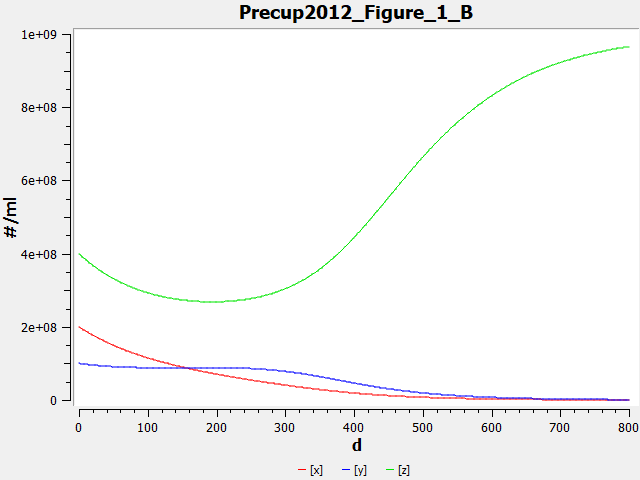## Precup2012 - Mathematical modeling of cell dynamics after allogeneic bone marrow transplantationModel Identifier
BIOMD0000000800
Short description
This is a basic mathematical model describing the dynamics of three cell lines (normal host cells, leukemic host cells and donor cells) after allogeneic stem cell transplantation.
Format
SBML (L2V4)
Related Publication
• Mathematical modeling of cell dynamics after allogeneic bone marrow transplantation• Precup, R., Arghirescu, S., Cucuianu, A., Erban, M.
• International Journal of Biomathematics , 3/ 2012 , Volume 5 , Issue 2 , DOI: 10.1142/S1793524511001684
• Department of Applied Mathematics, BabeBolyai University, Cluj 400084, Romania
• In this paper a basic mathematical model is introduced to describe the dynamics of three cell lines after allogeneic stem cell transplantation: normal host cells, leukemic host cells and donor cells. Their evolution is one of competitive type and depends upon kinetic and cellcell interaction parameters. Numerical simulations prove that the evolution can ultimately lead either to the normal hematopoietic state achieved by the expansion of the donor cells and the elimination of the host cells, or to the leukemic hematopoietic state characterized by the proliferation of the cancer line and the suppression of the other cell lines. One state or the other is reached depending on cellcell interactions (anti-host, anti-leukemia and anti-graft effects) and initial cell concentrations at transplantation. The model also provides a theoretical basis for the control of post-transplant evolution aimed at the achievement of normal hematopoiesis.
Contributors
Submitter of the first revision: Johannes Meyer
Submitter of this revision: Tung Nguyen
Modellers: Tung Nguyen, Johannes Meyer

is (2 statements)
BioModels Database MODEL1908190003
BioModels Database BIOMD0000000800

hasProperty (3 statements)
Mathematical Modelling Ontology Ordinary differential equation model
NCIt NCIT:C46089
NCIt Leukemia

isDescribedBy (1 statement)
isDerivedFrom (1 statement)

Curation status
Curated

Tags

#### Connected external resources

SBGN view in Newt Editor

Name Description Size Actions

### Model files

Precup2012.xml SBML L2V4 Representation of Precup2012 - Mathematical modeling of cell dynamics after allogeneic bone marrow transplantation 31.22 KB Preview | Download

Precup2012.cps COPASI file of Precup2012 - Mathematical modeling of cell dynamics after allogeneic bone marrow transplantation 61.40 KB Preview | Download
Precup2012.sedml SED-ML file of Precup2012 - Mathematical modeling of cell dynamics after allogeneic bone marrow transplantation 2.67 KB Preview | Download
• Model originally submitted by : Johannes Meyer
• Submitted: Aug 19, 2019 4:21:58 PM
##### Revisions

(*) You might be seeing discontinuous revisions as only public revisions are displayed here. Any private revisionsof this model will only be shown to the submitter and their collaborators.

Legends
: Variable used inside SBML models

Species
Species Initial Concentration/Amount
x

hematopoietic stem cell ; bone marrow
2.0E8 item
z

hematopoietic stem cell ; bone marrow
4.0E8 item
y

leukemic stem cell ; bone marrow
1.0E8 item
Reactions
Reactions Rate Parameters
x => compartment*c*x c = 0.01
z => compartment*c*z c = 0.01
=> y; x, z compartment*A/(1+B*(x+y+z))*(x+y+epsilon)/(x+y+epsilon+G*z)*y epsilon = 1.0; A = 0.45; B = 2.2E-8; G = 2.0
=> z; x, y compartment*a/(1+b*(x+y+z))*(1-h*(x+y)/(z+epsilon+h*(x+y)))*z epsilon = 1.0; b = 2.2E-8; h = 2.0; a = 0.23
y => compartment*C*y C = 0.01
=> x; y, z compartment*a/(1+b*(x+y+z))*(x+y+epsilon)/(x+y+epsilon+g*z)*x epsilon = 1.0; b = 2.2E-8; a = 0.23; g = 2.0Curator's comment:
(added: 19 Aug 2019, 16:21:47, updated: 19 Aug 2019, 16:21:47)
Reproduced plot of Figure 1B in the original publication. Parameters and initial conditions are as indicated in the text and figure caption, respectively. Model simulated and plot produced using COPASI 4.24 (Build 197).